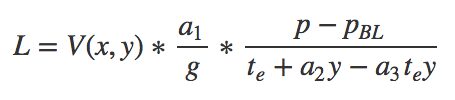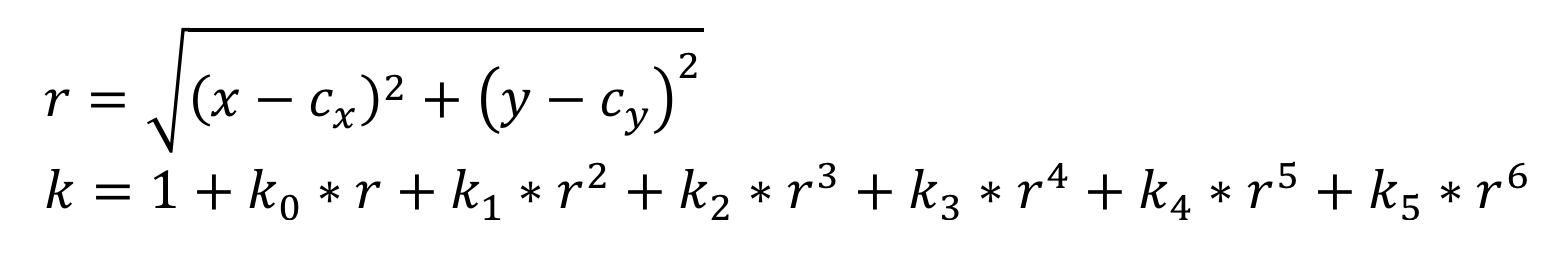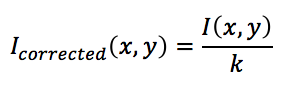# Radiometric Calibration Model for MicaSense Sensors

For users and partners that would like to implement their own raw image processing workflow software, we have published a series of tutorials and software on the use of RedEdge imagery and metadata for achieving the best results.  You can find the tutorials and code in github here: MicaSense Image Processing.

NOTE: This Radiometric Calibration model applies to all MicaSense sensors.

Relevant Camera Software Versions

The information on this page is applicable only for RedEdge software versions 2.1.0 and later.  If you have captured imagery with an older software version and would like to apply this calibration method to that imagery, please follow this procedure:

• cycle power on the camera and capture an image with the new firmware
• store the parameters from that image for use when processing images taken with older versions

The MicaSense radiometric calibration converts the raw pixel values of an image into absolute spectral radiance values, with units of W/m2/sr/nm. It compensates for sensor black-level, the sensitivity of the sensor, sensor gain and exposure settings, and lens vignette effects. All of the parameters used in the model can be read from the XMP metadata inside the TIFF file saved by the RedEdge camera; see Reading the Model Parameters from Metadata section below.

The formula for computing the spectral radiance L from pixel value p, is:Where,

• p is the normalized raw pixel value
• pBL is the normalized black level value (can be found in metadata tags)
• a1 a2, a3 are the radiometric calibration coefficients
• V(x, y) is the vignette polynomial function for pixel location (x, y). See “Vignette Model” section.
• te is the image exposure time
• g is the sensor gain setting (can be found in metadata tags)
• x, y are the pixel column and row number, respectively
• L is the spectral radiance in W/m2/sr/nm

Pixel Value Normalization

MicaSense sensors can save images in a 12-bit or 16-bit format. The radiometric model uses a normalized pixel value (p), in the range 0 to 1. To compute the normalized pixel value, simply divide the raw digital number for the pixel by 2^N, where N is the number of bits in the image. For 16-bit images, divide by 65536. For 12-bit images, divide by 4096. This applies to both the pixel value and the black level value.

Vignette Model

The RedEdge uses a radial vignette model to correct for the fall-off in light sensitivity that occurs in pixels further from the center of the image. To apply the model, first read cx, cy, and the six polynomial coefficients from the image metadata, then compute the formula below to find a correction scale factor for each pixel intensity.Where,

• r is the distance of the pixel (x,y) from the vignette center, in pixels
• (x,y) is the coordinate of the pixel being corrected
• k is the correction factor by which the raw pixel value should be divided to correct for vignette
• I(x,y) is the original intensity of pixel at x,y
• Icorrected(x,y) is the corrected intensity of pixel at x,y

In the radiance calculation above, V(x,y) is equal to 1/k

The parameters required for radiometric correction of the image are stored in the metadata inside each image. They can be read using standard tools such as the open-source packages exiv2 or exiftool.

Exposure and Gain Settings

The exposure time and gain are stored in the standard TIFF tags Exif.Image.ExposureTime and Exif.Photo.ISOSpeed. The gain is equal to the value of the ISOSpeed tag divided by 100.

Example Exiv2 command:

`exiv2 -K Exif.Photo.ISOSpeed -K Exif.Photo.ExposureTime -pa IMG_0000_1.tif`

Example Exiftool command:

`exiftool -ISOSpeed -ExposureTime IMG_0000_1.tif`

Example Exiftool response:

`ISO Speed : 100Exposure Time : 1/635`

Black Level Offset

The black level (pBL) is read from the standard TIFF tag Exif.Image.BlackLevel. This tag encodes an array of four values, which should be averaged to compute a black level offset which can be applied to all pixels.

Example Exiv2 command:

`exiv2 -K Exif.Image.BlackLevel -pa IMG_0000_1.tif`

Example Exiftool command:

`exiftool -BlackLevel IMG_0000_1.tif`

Example Exiftool response:

`Black Level : 4800 4800 4800 4800`

The three radiometric calibration parameters can be read from the XMP tag Xmp.MicaSense.RadiometricCalibration. The three coefficients are stored as an array, ordered as (ak1, ak2, ak3).

Example Exiv2 command:

`exiv2 -K Xmp.Micasense.RadiometricCalibration –px IMG_0000_1.tif`

Example Exiftool command:

`exiftool -RadiometricCalibration IMG_0000_1.tif`

Example Exiftool response:

`Radiometric Calibration : 9.3456090000000001e-05, 8.0701840000000006e-08, 1.8831690000000001e-05`

Vignette calibration

The eight parameters for the Vignette Model are stored in two XMP tags. The vignette center (cx, cy) is stored in Xmp.Camera.VignettingCenter. The array of polynomial coefficients, [k0, k1, k2, k3, k4, k5], is stored in Xmp.Camera.VignettingPolynomial.

Example Exiv2 command:

`exiv2 -K Xmp.Camera.VignettingPolynomial -K Xmp.Camera.VignettingCenter –px IMG_0000_1.tif`

Example Exiftool command:

`exiftool -VignettingPolynomial -VignettingCenter IMG_0000_1.tif`

Example Exiftool response:

`Vignetting Polynomial : 1.0021699999999999e-06, 5.5441760000000004e-07, -4.6130930000000004e-09, 1.207961e-11, -1.5006889999999999e-14, 6.7875920000000001e-18Vignetting Center : 607.53449999999998, 471.59350000000001`